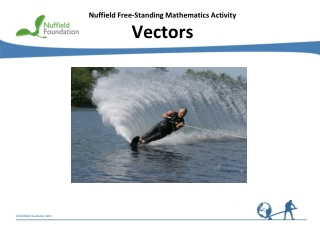DownloadDownload PresentationNuffield Free-Standing Mathematics Activity Vectors

# Nuffield Free-Standing Mathematics Activity Vectors

Download Presentation## Nuffield Free-Standing Mathematics Activity Vectors

- - - - - - - - - - - - - - - - - - - - - - - - - - - E N D - - - - - - - - - - - - - - - - - - - - - - - - - - -
##### Presentation Transcript

1. Vectors • Is the water skier moving in the same direction as the rope? • What forces are acting on the water skier? • Which directions are the forces acting in?

2. Vectors Scalar quantities have magnitude but no direction distance speed temperature Examples mass Vectorshave magnitude anddirection displacement velocity acceleration Examples force momentum

3. v = Unit vectors Suppose the velocity of a yacht has an easterly component of 12 ms–1 and a northerly component of 5 ms–1 The velocity isvms–1 wherev= 12i+ 5j irepresents a unit vectorto the east and jrepresents a unit vectorto the north Column vector notation

4. v  v= tan–1 Magnitude and direction of a vector v = v2 v1 Think about How can you use the triangle to find the magnitude and direction of v? Magnitude tan = Direction  =

5. N bearing v 5  12 = v= Example Think about How can you find the speed and direction of the yacht? v= = 13 Speed tan  = Direction = 22.6  The yacht is sailing at 13 ms–1 on bearing 067 (nearest )

6. To add or subtract vectors Add or subtract the components Example Forces acting on an object (in newtons) wherei is a horizontal unit vector to the right andjis a vertical unit vector upwards Think about how to find the total force Total force acting on the object

7. Example Displacements = (in metres) s 3s To multiply a vector by a scalar Multiply each component by the scalar Think about What do you get if you multiply both components of the vector by 3? 3s = Multiplying by 3 gives a displacement 3 times as big in the same direction

8. Equation 1 where u = initial velocity v= final velocity a = acceleration Equation 2 t= time taken Equation 3 s = displacement Constant acceleration equations mv Momentum is a vector

9. Forces and acceleration F1 Resultant force is the sum of the forces acting on a body, in this case F1 + F2 + F3 F2 F3 Newton’s First Law A particle will remain at rest or continue to move uniformly in a straight line unless acted upon by a non-zero resultant force. Newton’s Second Law F = ma Resultant forcecauses acceleration Newton’s Third Law Action and reaction are equal and opposite. This means if a body A exerts a force on a body B, then B exerts an equal and opposite force on A.

10. vC= vR= Speed N bearing 2.9  tan = 0.6 vR vS= Swimmer Find the magnitude and direction of the swimmer’s resultant velocity. Resultant velocity vR= (ms–1) i= unit vectorto the eastj= unit vectorto the north = 2.96 ms–1 = 0.2068 … Direction  = 11.7 The swimmer will travel at 2.96 ms–1 on bearing 102 (nearest )

11. O a u= a = v (ms–1) c s (m) Golf ball Find a the velocity at time t b the velocity when t= 2 c the ball’s displacement from O, when t = 2 i= horizontal unit vectorj= vertical unit vector (ms–1) b When t = 2 (m) When t = 2

12. b) = 60a u = F= a = Skier a Find the skier’s acceleration. b Find the speed and direction of the skier 20 seconds later. 60 kg i= unit vectorto the eastj= unit vectorto the north a)F = ma (ms–2) (ms–1) The skier is travelling at 1 ms–1 to the north.

13. u = R = Ship Ship travels at a constant velocity u ms–1 a What is the force, F, from the tug? b Ship’s initial position vector r i Find the position vector of the boat at time t. ii The ship is aiming for a buoy which has position vector Assuming the ship reaches the buoy, find x.

14. u = R = F = r O Ship a Ship travels at a constant velocity u ms–1 This means there is no acceleration b i Displacement Ship’s initial position vector r At time t, When ship reaches b ii 500 – t = 100 t = 400 x = 300 + 2.5t = 300 + 2.5 400 = 1300

15. Reflect on your work • How have you used the fact that i and jare perpendicular unit vectors? • Are there any similarities between the problems or the techniques you have used? • Can you think of other scenarios which could be tackled using vectors in component form?